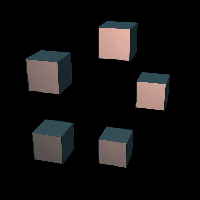# instance

Keywords:
• copy
• instance
• iterate
• repeat

Repeats its input some number of times, exposing the index as the iteration x value, and combines the results.

It is important to note that unless something in the input chain is making use of the iteration to change or move those copies, they will all be in the same position. The `rangeTransform` operator is designed for this purpose, though there are also other ops that can do so.

Refer to the Iteration guide for details.

It is also important to note that this operator, like `radialClone` evaluates its input separately for each iteration, which can cause a significant drain on resources if the input network is complex or costly.

## Parameters

• `Enable`:
• `Instance Count`: The number of copies to produce and merge.
• `Combine`: How to combine the copies. Only the "simple" options are guaranteed to work properly. The others may produce unexpected and problematic results.
• `Simple Union`: Combines the shapes so that all of their volumes are included.
• `Simple Intersect`: Combines the shapes so that only the places where all overlap are included.
• `Simple Difference`: Subtracts the second copy from the first, the third from that, and so on.
• `Smooth Union`:
• `Smooth Intersect`:
• `Smooth Difference`:
• `Round Union`:
• `Round Intersect`:
• `Round Difference`:
• `Chamfer Union`:
• `Chamfer Intersect`:
• `Chamfer Difference`:
• `Stair Union`:
• `Stair Intersect`:
• `Stair Difference`:
• `Column Union`:
• `Column Intersect`:
• `Column Difference`:
• `Radius`:
• `Number`:
• `Offset`:
• `Enable Transform`:
• `Transform CHOP`:
• `Enable Translate`:
• `Enable Rotate`:

## Inputs

• `definition_in`: (required)
• Coordinate Types: `float` `vec2` `vec3`
• Context Types: `Context` `MaterialContext` `CameraContext` `LightContext` `RayContext` `ParticleContext`
• Return Types: `Sdf`
• `Radius Field`: (optional)
• Coordinate Types: `float` `vec2` `vec3`
• Context Types: `Context` `MaterialContext` `CameraContext` `LightContext` `RayContext` `ParticleContext`
• Return Types: `float` `Sdf`

## Variables

• `index`:
• `normindex`: